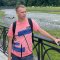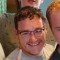# getCollectionGraphLast updated Dec 6th, 2019 | Page history | Improve this page | Report an issue

## Overview¶

`getCollectionGraph` allows you to automatically load up related objects by specifying a JSON style hash to its second argument (in other words, it automatically joins a table on its related tables). It's possible to nest the JSON hash so you also retrieve the related objects of the related objects, for example:

``````\$blogpost = \$modx->getCollectionGraph('BlogPost', '{ "Comments":{} }', 34 );
foreach ( \$blogpost->Comments as \$c ) {
/* ...do something with each comment... */
}
// OR, joining on related objects of related objects
\$TFR = \$modx->getCollectionGraph('TrackingformsResources', '{ "Resources":{ "MassUnit":{}, "VolumeUnit":{} } }', 123 );
``````

xPDO translates this all into the necessary database query that joins on the appropriate tables (e.g. perhaps named trackingforms_resources, resources, and units) – like most of the xPDO methods, you refer to a model's tables/classes via their aliases.

You can NOT set a limit on a getCollectionGraph when using an xPDOQuery object - you will be limiting the total number of rows fetched, which is not the same as the total number of top level (eg BlogPost) objects. You can get very unexpected results by doing so.

## Sample Snippet¶

The below example that specifies several related objects. In the Zip XML schema, there would be some sort of aggregate relationship defined for "TZ", "ST", and "CT".

``````<?php
\$out = false;
\$xpdo->setPackage('sw_zipCode', MODX_BASE_PATH.'wsw/model/', 'sw_');
\$collection= \$xpdo->getCollectionGraph('Zip', '{"TZ":{},"ST":{},"CT":{}}', \$lookupZip);
foreach (\$collection as \$obj)
{
if (is_object(\$obj))
{
\$out = \$obj->toArray();                      // 'Zip'
\$out[timezone] = \$obj->TZ->get('tzname');
\$out[state] = \$obj->ST->get('statename');
\$out[county] = \$obj->CT->get('countyname');
}
}
return \$out;
?>
``````

Note that when you are using `\$xpdo->newQuery()` to filter the results and have multiple field names which are the same, for example an "id" field, in one or more of the different classes you join, xPDO will fail to return any result. Simply prefix your fieldname with the classname in that case, for example myClassName.id

### Snippet Call¶

``````[[!zipCollectionGraph?lookupZip=`32117`]]
``````

### Output¶

``````Array
(
[id] => 32117
[city] => Daytona Beach
[areacode] => 386
[lat] => 29.233
[lon] => -81.0479
[sw_county_id] => 1800
[sw_states_id] => 15
[sw_timezones_id] => 4
[timezone] => Eastern
[state] => Florida
[county] => Volusia
)
``````

### Equivalent MySQL¶

``````\$xpdo->setPackage('sw_zipCode', MODX_BASE_PATH.'wsw/model/', 'sw_');
\$collection= \$xpdo->getCollectionGraph('Zip', '{"TZ":{},"ST":{},"CT":{}}', \$lookupZip);
``````

In MySQL:

``````SELECT *
FROM `sw_zips` AS Z
LEFT JOIN `sw_county` AS CT ON CT.`id` = Z.`sw_county_id`
LEFT JOIN `sw_states` AS ST ON ST.`id` = Z.`sw_states_id`
LEFT JOIN `sw_timezones` AS TZ ON TZ.`id` = Z.`sw_timezones_id`
WHERE Z.`id` = 32117
``````

### The Schema¶

The following schema is greatly simplified for readability and this example:

Though a functional schema, this is not a final schema by any stretch of the imagination.

``````<model package="sw_zipCode" version="1.0" baseClass="xPDOObject" platform="mysql" defaultEngine="MyISAM">
<object class="class="City" table="city" extends="xPDOSimpleObject">
<field key="cityname" dbtype="varchar" precision="50" phptype="string" null="false"/>
</object>
<object class="Cityzip" table="cityzip" extends="xPDOSimpleObject">
<field key="city" dbtype="int" precision="10" phptype="integer" null="false"/>
<field key="zip" dbtype="int" precision="5" phptype="integer" null="false"/>
</object>
<object class="County" table="county" extends="xPDOSimpleObject">
<field key="countyname" dbtype="varchar" precision="35" phptype="string" null="true" index="index"/>
</object>
<object class="States" table="states" extends="xPDOSimpleObject">
<field key="statename" dbtype="varchar" precision="40" phptype="string" null="false" index="index"/>
<field key="abbrv" dbtype="char" precision="2" phptype="string" null="false"/>
</object>
<object class="Timezones" table="timezones" extends="xPDOSimpleObject">
<field key="tzname" dbtype="varchar" precision="20" phptype="string" null="true" index="index"/>
</object>
<object class="Zip" table="zips" extends="xPDOSimpleObject">
<field key="city" dbtype="varchar" precision="50" phptype="string" null="true"/>
<field key="areacode" dbtype="int" precision="3" phptype="integer" null="true"/>
<field key="lat" dbtype="float" phptype="float" null="true"/>
<field key="lon" dbtype="float" phptype="float" null="true"/>
<field key="sw_county_id" dbtype="int" precision="4" phptype="integer" null="false" index="pk"/>
<field key="sw_states_id" dbtype="int" precision="2" phptype="integer" null="false" index="pk"/>
<field key="sw_timezones_id" dbtype="int" precision="2" phptype="integer" null="false" index="pk"/>

<aggregate alias="TZ" class="Timezones" local="tz_id" foreign="id" cardinality="one" owner="foreign" />
<aggregate alias="ST" class="County" local="sw_county_id" foreign="id" cardinality="one" owner="foreign" />
<aggregate alias="CT" class="States" local="sw_states_id" foreign="id" cardinality="one" owner="foreign" />
</object>
</model>
``````

## Another Example¶

Another relation example that is common is joining MODX pages with their Template Variable values. Sometimes this does not work as expected since values are stored differently than you might expect. But here's a walk-through.

``````\$pages = \$modx->getCollectionGraph('modResource', '{"TemplateVarResources":{}}', array('parent'=>12));

foreach (\$pages as \$p) {
foreach (\$p->TemplateVarResources as \$tv) {
// Do stuff here with the TV
\$tv_array = \$tv->toArray();
\$tv->get('value');
}
}
``````

1. Obtain a connection via the xPDO Constructor including Hydrating Fields
2. Viewing the package name in the schema we set (or apply) the package to our connection, taking note of the prefix our tables are using in the database
3. Using 'Zip' as our "view" we look at the relationships directly defined in the Zip object, in our schema, and access those via the aliases given there

Everything is about the schema definition. A poorly thought out and developed schema may very well lead to many hours of frustration.

If you are having trouble with xPDO, you have two main avenues of troubleshooting:

• First and foremost -- the schema is not correct. Re thinking it from the bottom relations up, and through each relationship may help us "see" where we may be missing it.
• Not understanding what we are seeing is another huge issue.
• Understand the point of your schema. If your schema will eventually instantiate an object representing a single entity (such as a user) your base relationships should be (\$this->user) 1: 1 or many on the other side.
• A relationship tied to a many-to-many relation (as in the relations between users and groups) will probably need a for each loop to filter through the sub relation.
• Aggregate relations should typically be singular. Removing them does nothing to the related data
• Composite relations should typically be plural. Removing them also removes each of the related child relations.
• Don't be afraid to use regular language in your schema. Instead of Cityzip, in the schema above, Cityhaszips might be a bit clearer in thinking through your schema
• Don't use the same class name in multiple places in the schema. Not only will it bring confusion while coding, I suspect it also confuses xPDO. If for no other reason -- its just bad form.
• xPDO is fast, very fast. If your queries are taking to long, go back to the schema and follow the indexes.
• xPDO likes primary keys, so build your relations around primary keys when ever possible -- if not always.
• In case you missed it '{"TZ":{},"ST":{},"CT":{}}' is JSON formatted.

Support the team building MODX with a monthly donation.

The budget raised through OpenCollective is transparent, including payouts, and any contributor can apply to be paid for their work on MODX.

Backers

•••••••••••••Budget

\$217 per month—let's make that \$500!